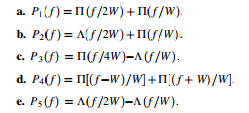Create an Account

Home / Questions / Sketch the following spectra and tell which ones satisfy Nyquist’s pulse shape criterion. ...

Sketch the following spectra and tell which ones satisfy Nyquist’s pulse shape criterion. For those that do, find the appropriate sample interval T in terms of W Find the corresponding pulse shape

Sketch the following spectra and tell which ones satisfy Nyquist’s pulse shape criterion. For those that do, find the appropriate sample interval, T, in terms of W. Find the corresponding pulse shape function p(t): (Recall that P(f) =Ais a unit-high rectangular pulse from -A/2 to A/2; L(ƒ =B) is a unit-high triangle from( -B to B.)Jul 31 2020 View more View LessSubscribe To Get Solution